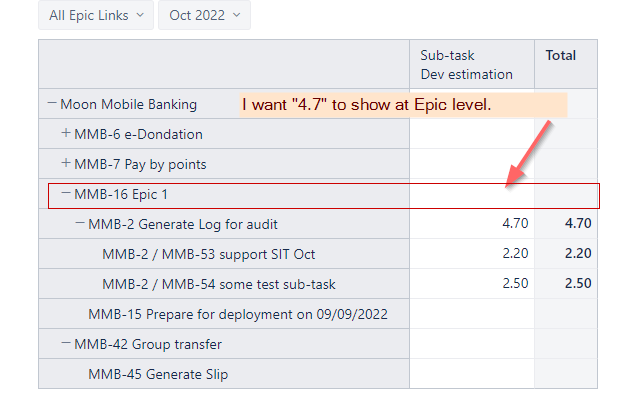# How to get sub-task for stories under epic

I have a custom field Dev estimation.
I would like the Epic level to show the sum of Dev estimation of sub-tasks of all stories under the epic.I tried this but have no luck.

``````CASE WHEN [Measures].[Issue type] = "Sub-task"  THEN
[Measures].[Issue Dev Estimation].Value

ELSE

Sum(
Filter( [Issue].[Issue].GetMembersByKeys(
,
DateInPeriod(
([Measures].[Transition to status last date]
,[Transition Status].[Done]),
[Time].CurrentHierarchyMember
)
AND
(
AND
[Measures].[Issue status] = "Done"
)

),[Measures].[Issue Dev Estimation])

END
``````

The aggregation stops at the Parent issue level because Epic does not have the property “Sub-task keys”.

If you only add the “Dev estimation” value to the sub-tasks, you might import it as a measure, which will automatically aggregate over the hierarchy.
Otherwise, if you have separate “Dev estimation” values for the Parent and Epic issues and you only want the values of sub-tasks, you might iterate through the Epic hierarchy and sum the values of relevant sub-tasks.

You might be able to do that with the following expression.

``````Sum(
Filter(
--set of underlying sub-tasks within the hierarchy
DescendantsSet([Issue].CurrentHierarchyMember,
--filtering conditions
[Measures].[Issue status] = "Done"
AND
--2)moved to done in the current report context
DateInPeriod(
([Measures].[Transition to status last date],
[Transition Status].[Done]),
[Time].CurrentHierarchyMember)
),
--value for sum
[Issue].CurrentHierarchyMember.Get('Dev Estimation')
)
``````

Regards,
Oskars / support@eazyBI.com

1 Like

Thank you. That works!!!

1 Like